# pie3

•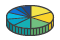## Syntax

``pie3(X)``
``pie3(X,explode)``
``pie3(X,labels)``
``pie3(X,explode,labels)``
``pie3(ax,___)``
``p = pie3(___)``

## Description

example

````pie3(X)` draws a three-dimensional pie chart using the data in `X`. Each slice of the pie chart represents an element in `X`.```

example

````pie3(X,explode)` specifies which slices to offset from the center of the pie chart.```

example

````pie3(X,labels)` specifies text labels for the slices. The number of labels must equal the number of elements in `X`.```
````pie3(X,explode,labels)` offsets slices and specifies the text labels.```

example

````pie3(ax,___)` plots into the axes specified by `ax` instead of into the current axes (`gca`). The argument `ax` can precede any of the input argument combinations in the previous syntaxes.```

example

````p = pie3(___)` returns a vector of the `Patch`, `Surface`, and `Text` objects that make up the pie chart. Use `p` to modify properties of the chart after creating it. For a list of properties you can set for these objects, see Patch Properties, Surface Properties, and Text Properties.```

## Examples

collapse all

Create a 3-D pie chart of vector X.

```X = [1 3 0.5 2.5 2]; pie3(X)```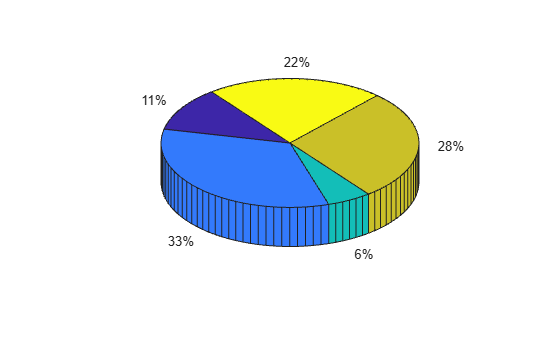Create a 3-D pie chart and offset a slice by specifying the corresponding `explode` element as 1.

```X = [1 3 0.5 2.5 2]; explode = [0 1 0 0 0]; pie3(X,explode)```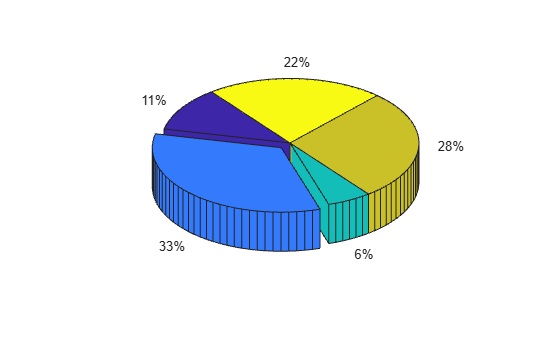Create a 3-D pie chart and specify the text labels.

```X = 1:3; labels = ["Taxes" "Expenses" "Profit"]; pie3(X,labels)```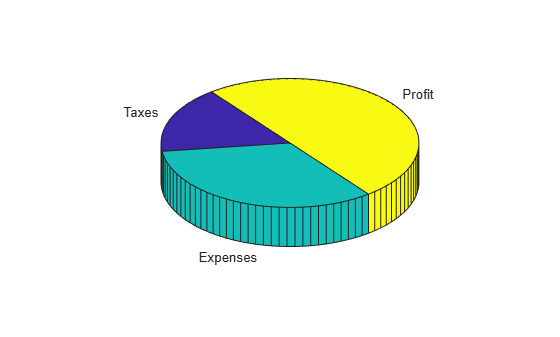Create a 3-D pie chart and modify the font style of one of the labels.

First, create a 3-D pie chart with the default font styling. Specify an output argument, `p`, so that you can use it to customize the pie chart.

```X = [1 2 3]; labels = ["Taxes" "Expenses" "Profit"]; p = pie3(X,labels);```Each slice in the pie chart has four corresponding elements in `p`: `Patch`, `Surface`, `Patch`, and `Text`.

`p'`
```ans = 12x1 graphics array: Patch Surface (Taxes) Patch Text (Taxes) Patch Surface (Expenses) Patch Text (Expenses) Patch Surface (Profit) Patch Text (Profit) ```

Get the `Text` object for the label `Expenses`. Change its color and font size by using dot notation to set the associated properties.

```t = p(8); t.Color = "red"; t.FontSize = 14;```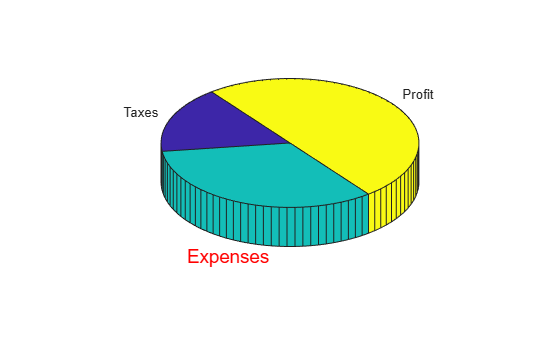Compare two 3-D pie charts by plotting them in the same figure using a tiled chart layout.

Create vectors of financial data for 2010 and 2011 and a set of labels.

```y2010 = [50 0 100 95]; y2011 = [65 22 97 120]; labels = ["Investments" "Cash" "Operations" "Sales"];```

Create a `2`-by-`1` tiled chart layout. Create a pie chart for 2010 in the first tile and for 2011 in the second tile. Add a shared legend for the pie charts.

```t = tiledlayout(1,2,"TileSpacing","None"); ax1 = nexttile; pie3(ax1,y2010) title("2010") ax2 = nexttile; pie3(ax2,y2011) title("2011") l = legend(labels); l.Layout.Tile = "south";```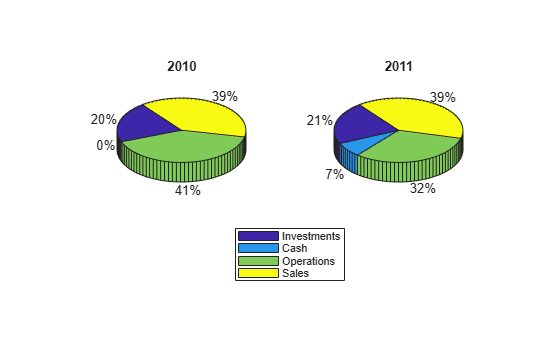## Input Arguments

collapse all

Input array, specified as a numeric vector or matrix. Each element of `X` corresponds to a slice of the pie chart. The sum, S, of all the elements in `X` determines how `pie3` displays the chart:

• If S = 1, then the values in `X` specify the proportions of the slices.

• If S < 1, then the values in `X` specify the proportions of the slices, and `pie3` draws a partial pie chart.

• If S > 1, then `pie3` normalizes the size of each slice by S.

Offset slices, specified as a numeric or logical vector or matrix the same size as `X`. To offset a slice, set the corresponding element to either a nonzero value or `true`. The value of a nonzero element does not impact the offset.

Example: `[0 1 0 0]` offsets the second slice.

Text labels for slices, specified as a string array or cell array of character vectors. If you do not specify labels, `pie3` automatically displays percentage values.

Target axes, specified as an `Axes` object. If you do not specify the axes, MATLAB® plots into the current axes or it creates an `Axes` object if one does not exist.

## Output Arguments

collapse all

Graphics objects that make up the pie chart, returned as a vector of `Patch`, `Surface`, and `Text` objects.

Each slice in the pie chart consists of four objects that you can use to modify the chart appearance. As a result, the length of the output vector is four times the number of elements in `X`. Each slice has four corresponding elements in `p` in this order:

• `Patch` object – Bottom layer (not visible in the image)

• `Surface` object – Surface between top and bottom layer (orange in the image)

• `Patch` object – Top layer (blue in the image)

• `Text` object – Text label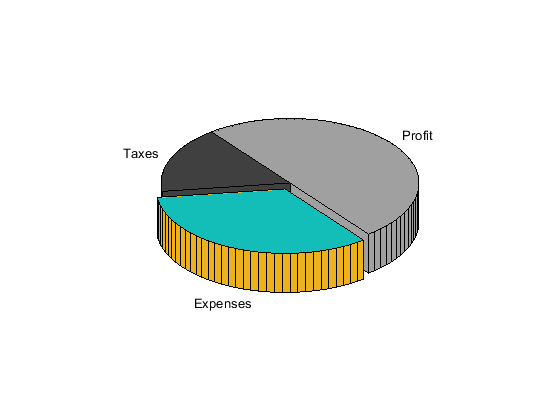For more information about the properties you can set for these objects, see Patch Properties, Surface Properties, and Text Properties.

## Version History

Introduced before R2006a

expand all